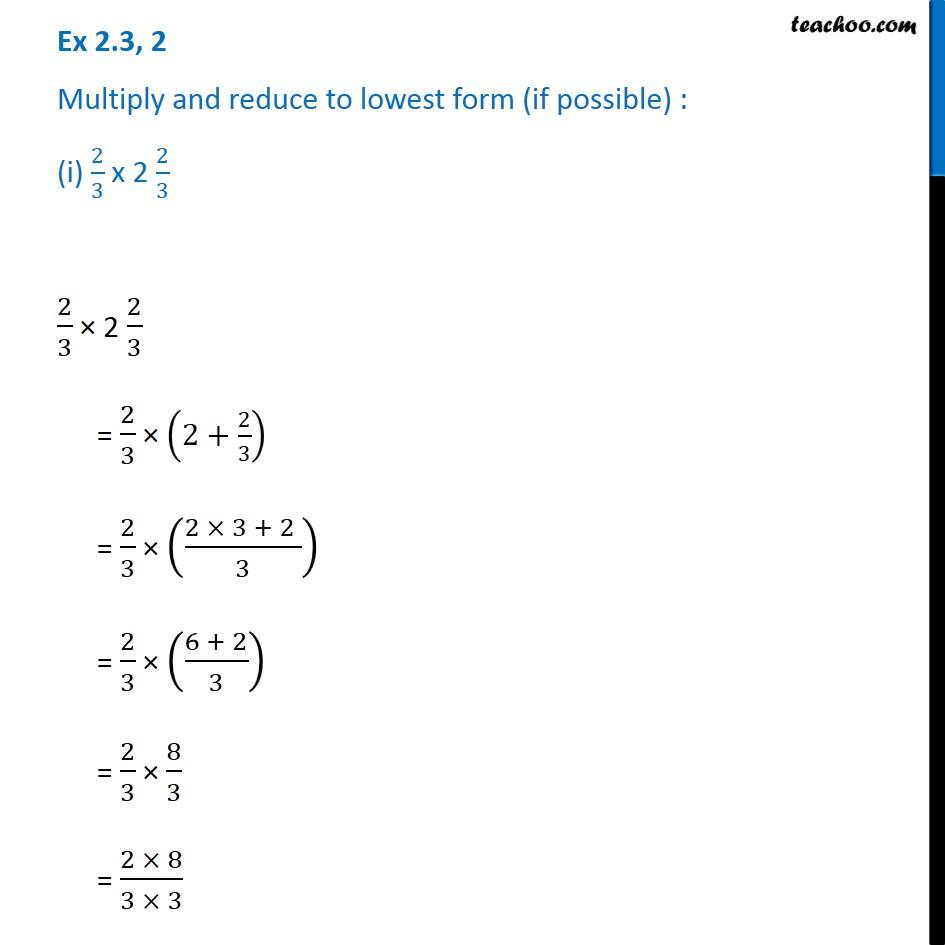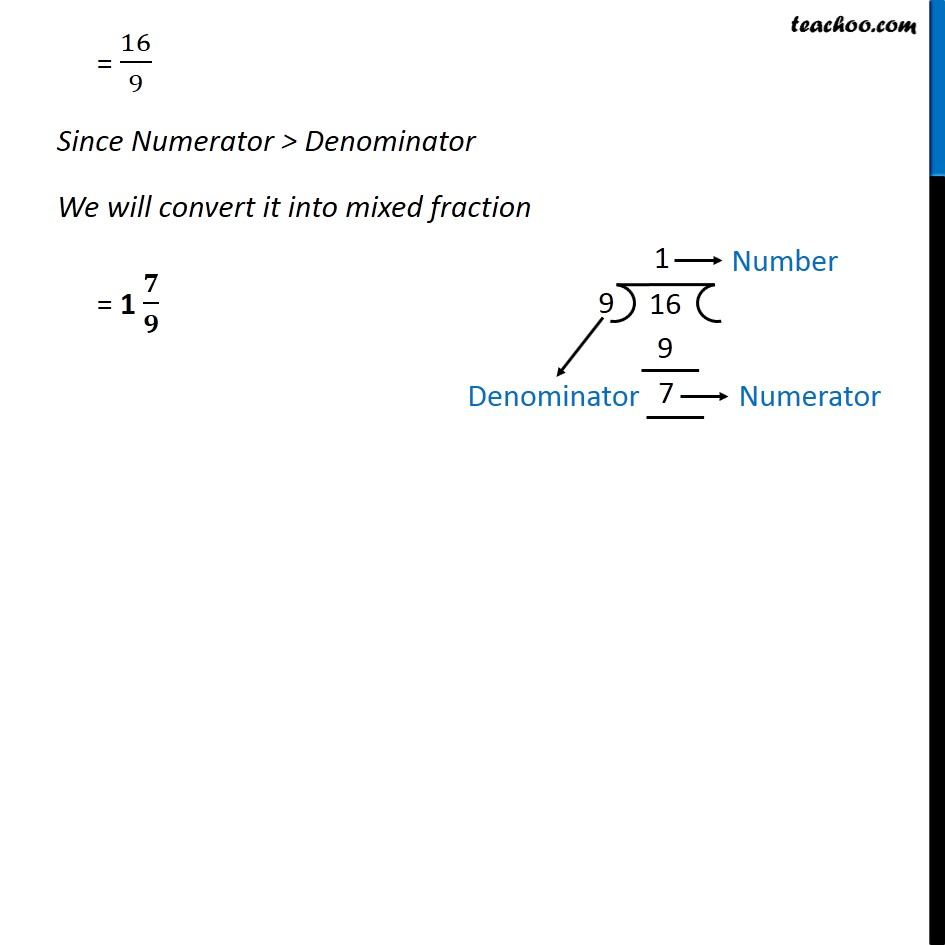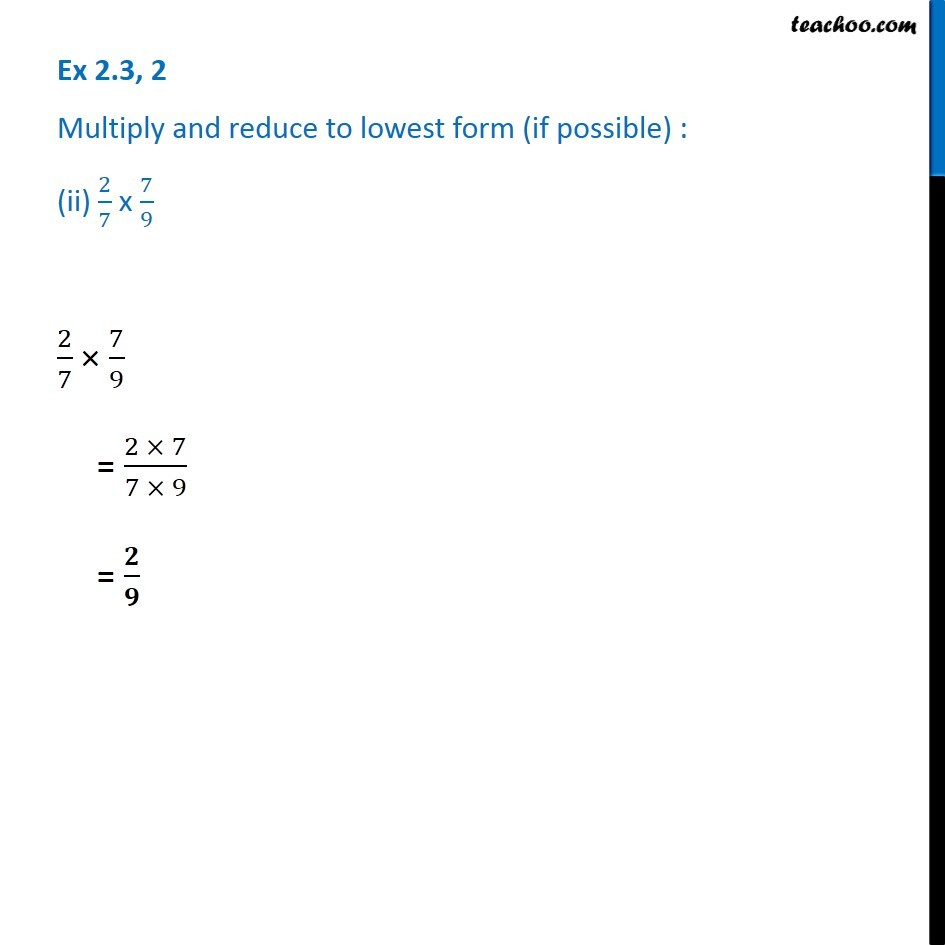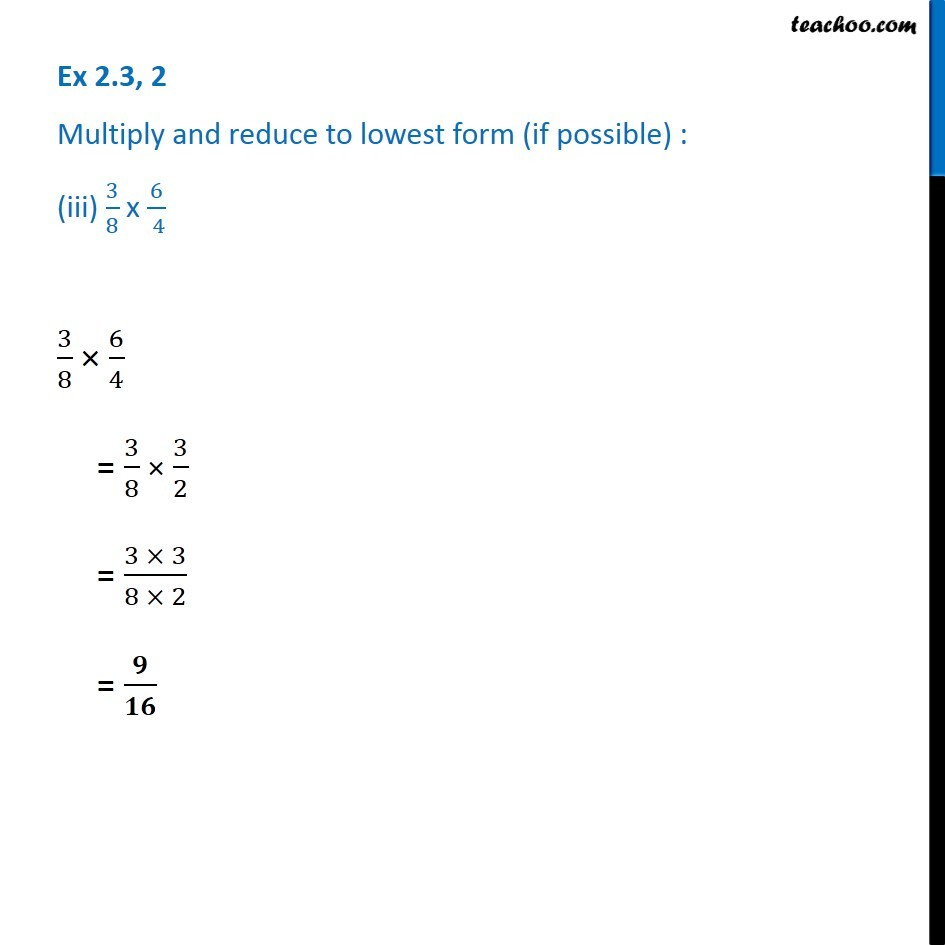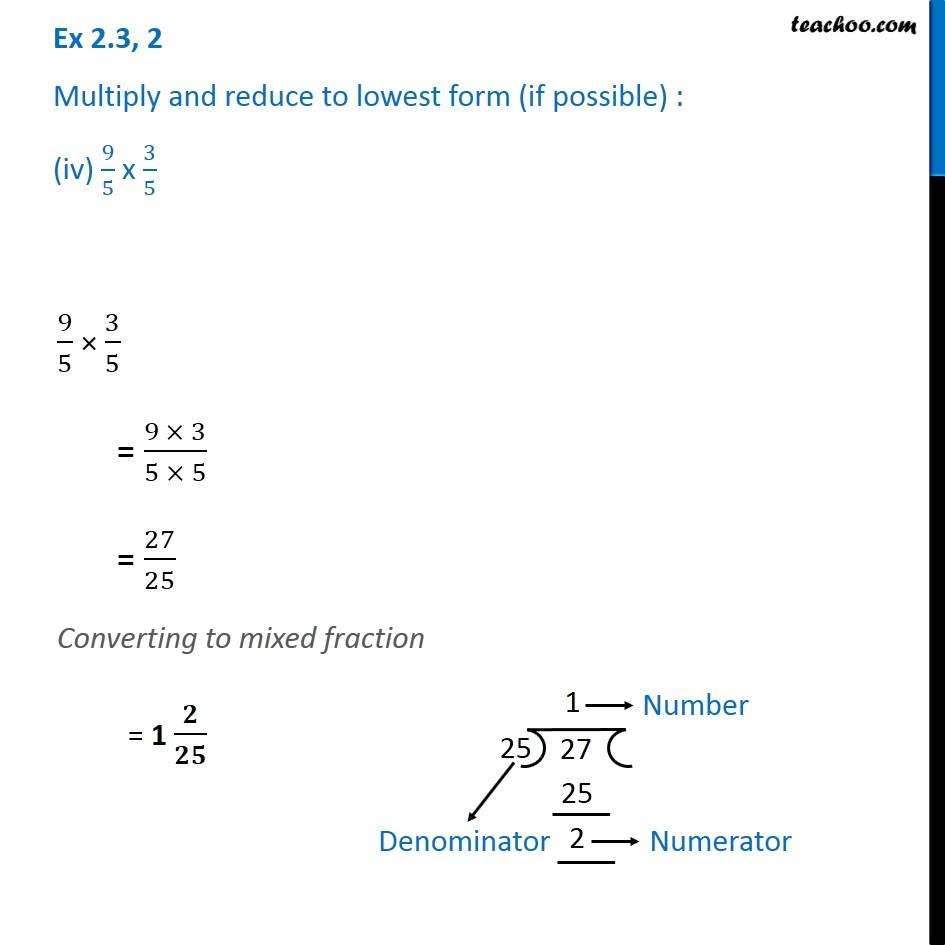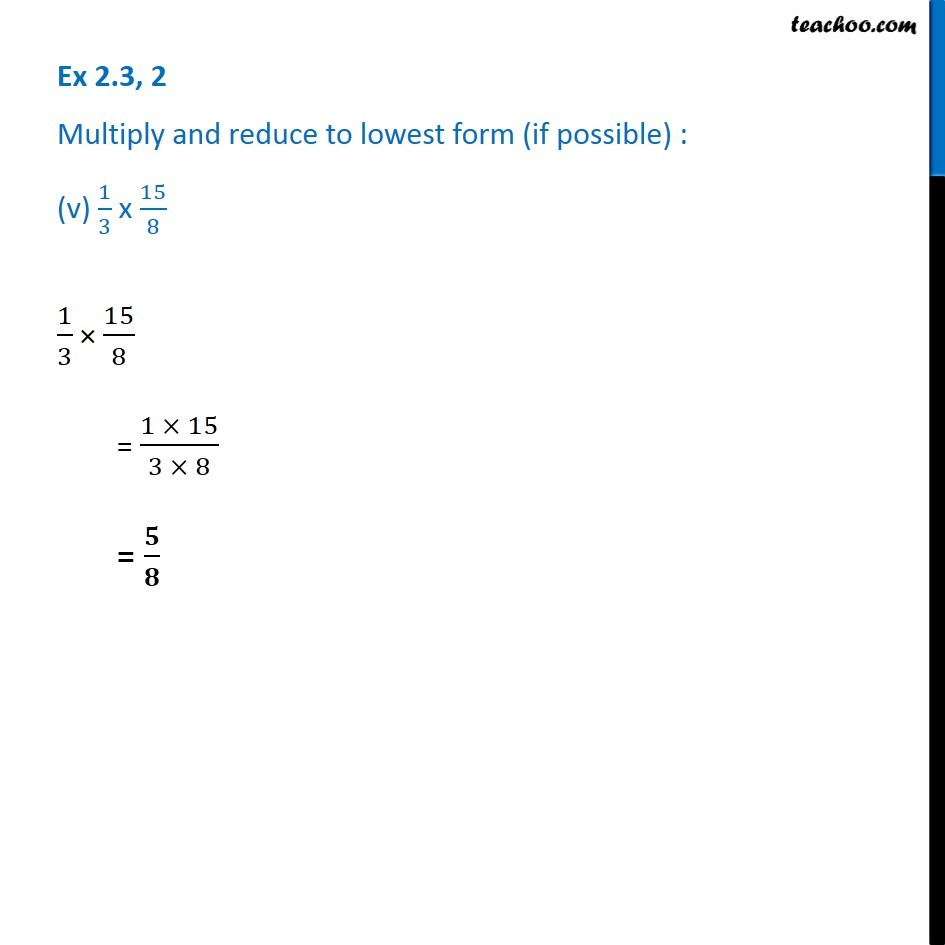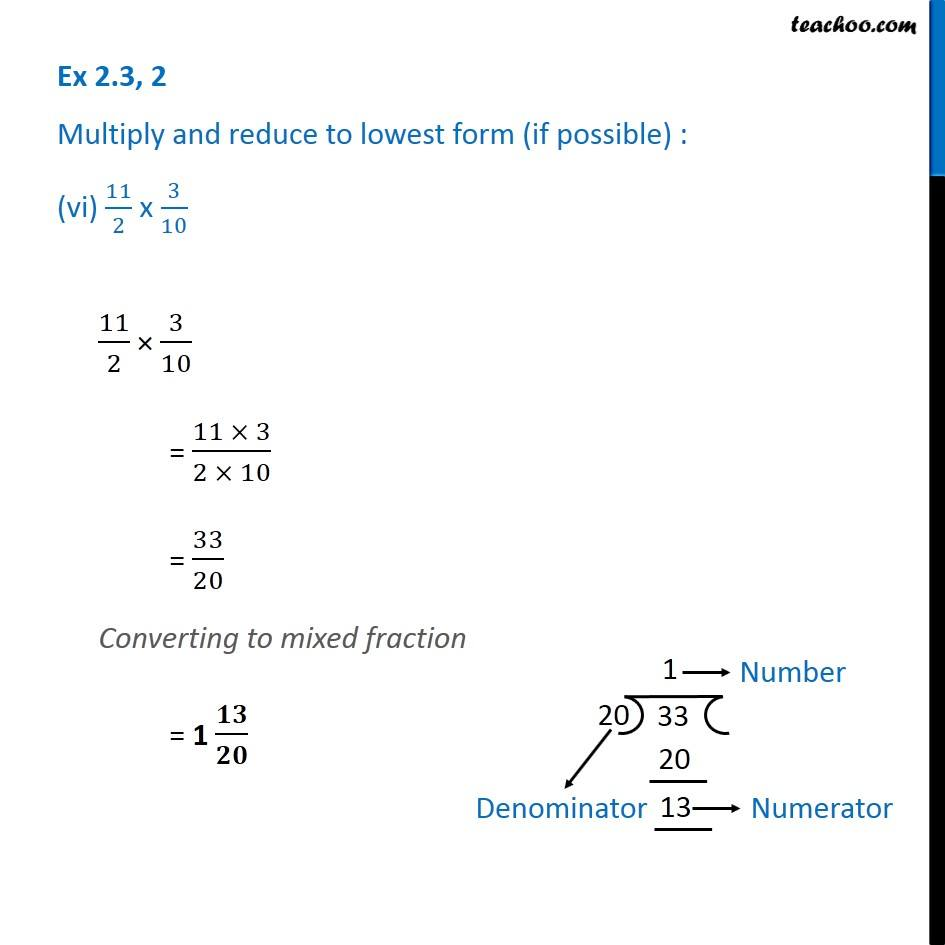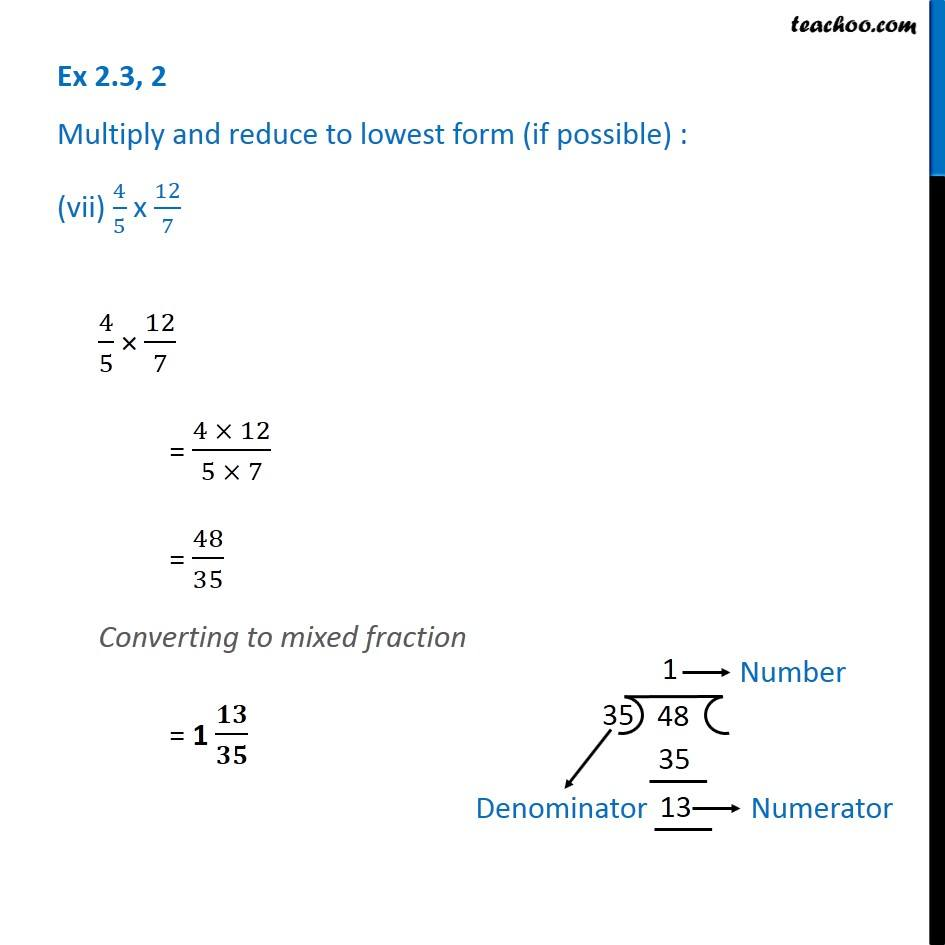Subscribe to our Youtube Channel - https://you.tube/teachoo

1. Chapter 2 Class 7 Fractions and Decimals
2. Serial order wise
3. Ex 2.3

Transcript

Ex 2.3, 2 Multiply and reduce to lowest form (if possible) : (i) 2/3 x 2 2/3 2/3 × 2 2/3 = 2/3 × (2+2/3) = 2/3 × ((2 × 3 + 2 )/3) = 2/3 × ((6 + 2)/3) = 2/3 × 8/3 = (2 × 8)/(3 × 3) = 16/9 Since Numerator > Denominator We will convert it into mixed fraction = 1 𝟕/𝟗 Ex 2.3, 2 Multiply and reduce to lowest form (if possible) : (ii) 2/7 x 7/9 2/7 × 7/9 = (2 × 7)/(7 × 9) = 𝟐/𝟗 Ex 2.3, 2 Multiply and reduce to lowest form (if possible) : (iii) 3/8 x 6/( 4) 3/8 × 6/4 = 3/8 × 3/2 = (3 × 3)/(8 × 2) = 𝟗/𝟏𝟔 Ex 2.3, 2 Multiply and reduce to lowest form (if possible) : (iv) 9/5 x 3/5 9/5 × 3/5 = (9 × 3)/(5 × 5) = 27/25 Converting to mixed fraction = 1 𝟐/𝟐𝟓 Ex 2.3, 2 Multiply and reduce to lowest form (if possible) : (v) 1/3 x 15/81/3 × 15/8 = (1 × 15)/(3 × 8) = 𝟓/𝟖 Ex 2.3, 2 Multiply and reduce to lowest form (if possible) : (vi) 11/2 x 3/10 11/2 × 3/10 = (11 × 3)/(2 × 10) = 33/20 Converting to mixed fraction = 1 𝟏𝟑/𝟐𝟎 Ex 2.3, 2 Multiply and reduce to lowest form (if possible) : (vii) 4/5 x 12/7 4/5 × 12/7 = (4 × 12)/(5 × 7) = 48/35 Converting to mixed fraction = 1 𝟏𝟑/𝟑𝟓

Ex 2.3